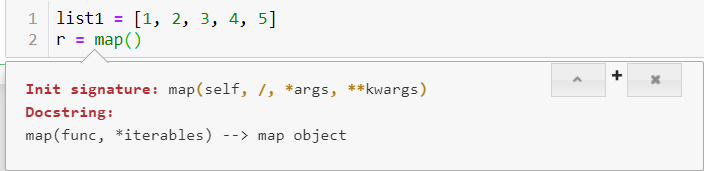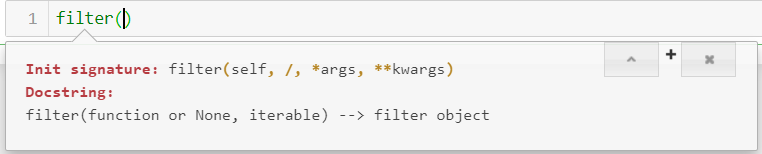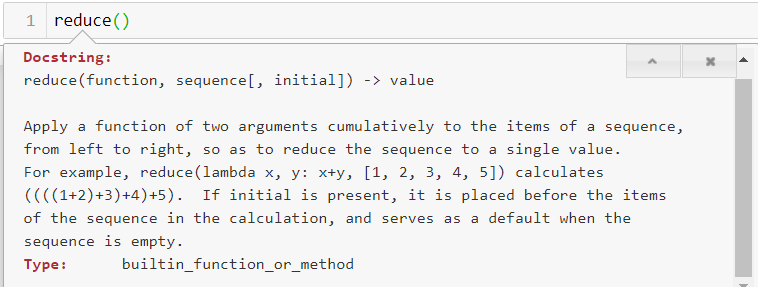python一些高级应用的再学习

### 1.lambda表达式

lambda表达式本质上其实就是python的匿名函数。并且也可以作为返回值返回一个匿名函数。这个在下面会具体说到。格式为 lambda 参数列表：函数体。

``````a = lambda x,y:x+y
a(3, 4)

return x+y

``````

### 2.三元运算符

``````condition = True
ans = 1 if condition else 2

condition = False
ans = 1 if condition else 2
``````

### 3.map函数``````list1 = [1, 2, 3, 4, 5]
r = map(lambda x:x+x, list1)
print(list(r))

``````

``````m1 = map(lambda x,y:x*x+y, [1, 2, 3, 4], [1, 2, 3, 4])
list(m1)

``````

### 4.filter过滤器``````def is_not_none(s):
return s and len(s.strip()) > 0
list1 = filter(is_not_none, ['hello', ' ', None, 'love'])
# 用lambda实现相同的效果
list2 = filter(lambda s:s and len(s.strip()) > 0, ['hello', ' ', None, 'love'])
print(list(list1))
print(list(list2))

['hello', 'love']
``````

### 5.reduce函数``````from functools import reduce
f = lambda x, y : x + y
reduce(f, [1, 2, 3, 4, 5])

f = lambda x, y : x + y
reduce(f, [1, 2, 3, 4, 5], 10)

``````

### 6.python中的三大推导式

#### 列表推导式

``````list1 = [1, 2, 3, 4, 5, 6]
f = map(lambda x: x + x, list1)
print(list(f))

list2 = [i+i for i in list1]
print(list2)
list3 = [i**3 for i in list1]
print(list3)

list4 = [i*i for i in list1 if i > 3]
print(list4)

[2, 4, 6, 8, 10, 12]
[2, 4, 6, 8, 10, 12]
[1, 8, 27, 64, 125, 216]
[16, 25, 36]
``````

#### 集合推导式

``````list1 = [1, 2, 3, 4, 5, 6]

list2 = {i+i for i in list1}
print(list2)
list3 = {i**3 for i in list1}
print(list3)

list4 = {i*i for i in list1 if i > 3}
print(list4)

{2, 4, 6, 8, 10, 12}
{64, 1, 8, 216, 27, 125}
{16, 25, 36}
``````

#### 字典推导式

``````s = {
"Alice": 10,
"Bob": 20,
"Jack": 30
}
# 拿出所有的key
s_key = [key for key, value in s.items()]
print(s_key)
s_key = [key+"xxx" for key, value in s.items()]
print(s_key)

# key和value颠倒
s_key_value = {value:key for key,value in s.items()}
print(s_key_value)

s_select = {key:value for key,value in s.items() if key == 'Alice'}
print(s_select)

['Alice', 'Bob', 'Jack']
['Alicexxx', 'Bobxxx', 'Jackxxx']
{10: 'Alice', 20: 'Bob', 30: 'Jack'}
{'Alice': 10}
``````

### 7.闭包

js中也有闭包，这两个是否类似我也不太清楚。python中的闭包一般指返回值为函数的函数。说的严谨一点：在一个外函数中定义了一个内函数，内函数里运用了外函数的临时变量，并且外函数的返回值是内函数的引用。这样就构成了一个闭包。

``````import time

# 调用后打印当前时间
def run_time():
def now_time():
print(time.time())
return now_time

f = run_time()
f()
# 等价于 run_time()()

1582780675.5656545
``````
``````# 找到文件中有关键字的行
def mak_filter(key_word):
def the_filter(file_name):
doc = [line.strip() for line in open(file_name) if key_word in line]
return doc
return the_filter

f = mak_filter('Internet')
ans = f('./data/xjbz.txt')
print(ans)
print(len(ans))

['GhostSurf Platinum ensures your safety online by providing an anonymous, encrypted Internet connection, and GhostSurf stops spyware,', 'options enable you to block personal information, mask your IP address, route your data through anonymous hubs and even encrypt your Internet', "connection. GhostSurf's Privacy Control Center allows you to see and block every piece of data that your computer emits over the Internet,", 'preventing even your Internet Service Provider (ISP) from creating a profile on you.']
4
``````

### 8.装饰器

``````# 获取函数运行时间
import time

def runtime(func):
def get_time():
# 注意这两行和上面的区别
func()
print(time.time())
return get_time

@runtime
def student_run():
print("学生跑")

student_run()

1582783099.6487942
``````

@其实是装饰器的一个语法糖，说明我们的student_run方法被runtime方法装饰了，并在runtime里面的gettime方法实现studentrun自己的功能，但是却可以实现一些自己不具有的通用的功能，如验证、打印日志等等，非常方便。但是如果函数有参数呢？我们再看这一个例子：

``````# 获取函数运行时间
import time

def runtime(func):
def get_time(i):
# 注意这两行和上面的区别
func(i)
print(time.time())
return get_time

@runtime
def student_run(i):
print("学生跑")

student_run(1)

1582793456.4579806
``````

``````# 获取函数运行时间
import time

def runtime(func):
def get_time(*args):
# 注意这两行和上面的区别
func(*args)
print(time.time())
return get_time

@runtime
def student_run(i):
print("学生跑")

student_run(1)

1582793786.913682
``````

``````# 获取函数运行时间
import time

def runtime(func):
def get_time(*args, **kwargs):
# 注意这两行和上面的区别
func(*args, **kwargs)
print(time.time())
return get_time

@runtime
def student_run(i):
print("学生跑")

@runtime
def student1_run():
print("学生1跑")

@runtime
def studeent2_run(*args, **kwargs):
print("学生2跑")

student_run(1)
student1_run()
studeent2_run(a=1, b=2)

1582795276.119493

1582795276.119493

1582795276.119493
``````

### 9.@staticmethod && @classmethod

``````class A(object):
def foo(self, x):
print("executing foo(%s, %s)"%(self, x))

@classmethod
def class_foo(cls, x):
print("executing class_foo(%s, %s)" % (cls, x))

@staticmethod
def static_foo(x):
print("executing static_foo(%s)" % x)

a = A()
a.foo(1)
a.class_foo(1)
A.class_foo(1)
a.static_foo(1)
A.static_foo(1)

executing foo(<__main__.A object at 0x000001FBA605EF60>, 1)
executing class_foo(<class '__main__.A'>, 1)
executing class_foo(<class '__main__.A'>, 1)
executing static_foo(1)
executing static_foo(1)
``````

Python：staticmethod 和 classmethod的比较

@staticmethod和@classmethod的用法

Python中的 staticmethod 和 classmethod

上一篇数据分析的王炸--numpy&&pandas(1)

2020-02-28Noisy Channel Model && How to estimate Language Model

2020-02-26
目录